# RD Sharma Class 10 Solutions Chapter 4 Quadratic Equations Ex 4.1

## RD Sharma Class 10 Solutions Chapter 4 Quadratic Equations Ex 4.1

These Solutions are part of RD Sharma Class 10 Solutions. Here we have given RD Sharma Class 10 Solutions Chapter 4 Quadratic Equations Ex 4.1

Other Exercises

Question 1.
Which of the following are quadratic equations ?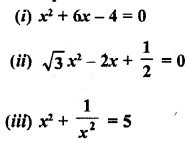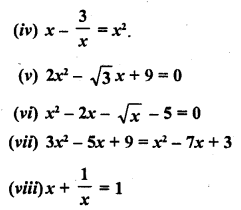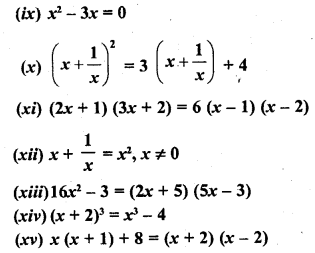Solution: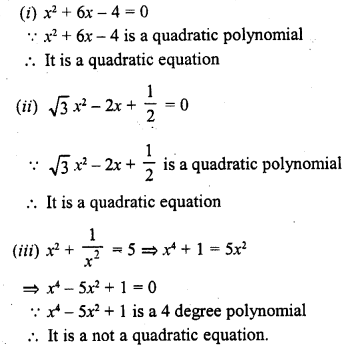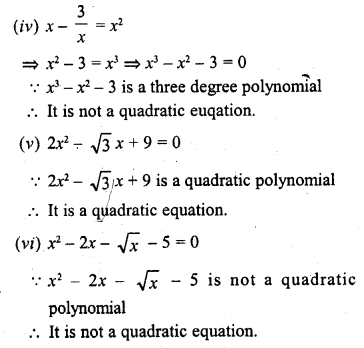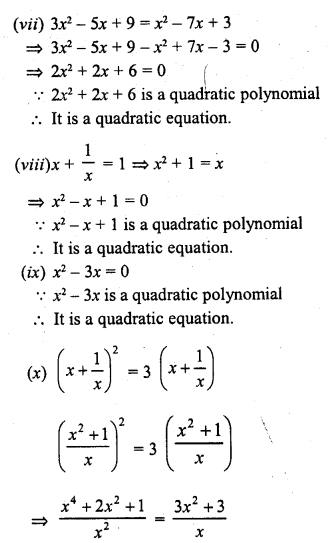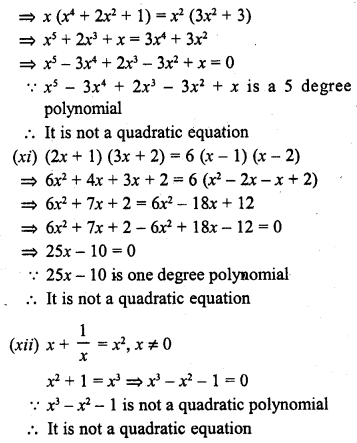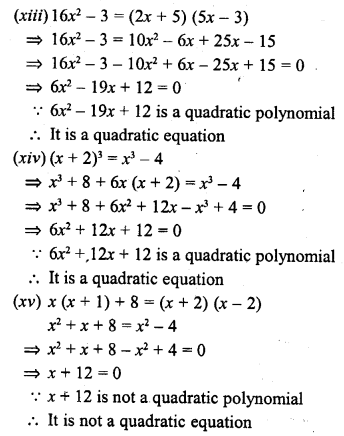Question 2.
In each of the following, determine whether the given values are solutions of the given equation or not :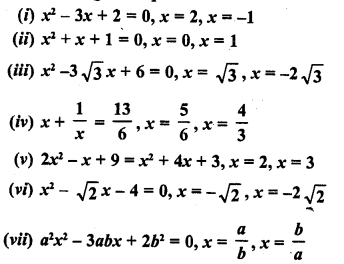Solution: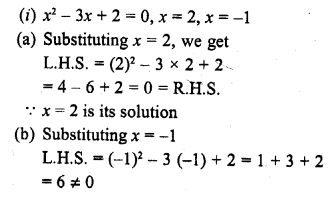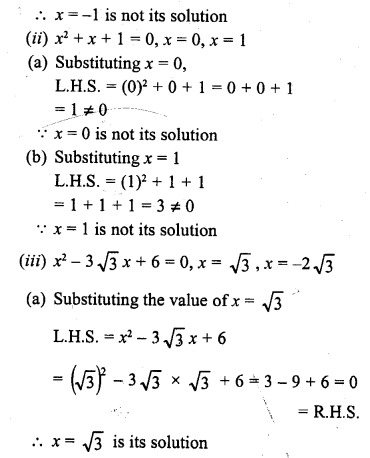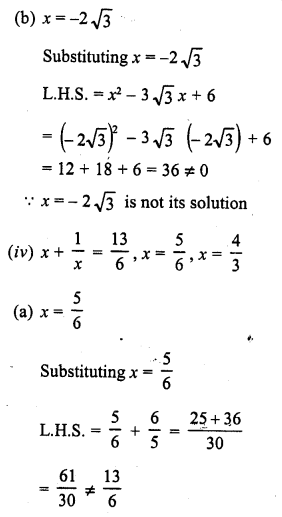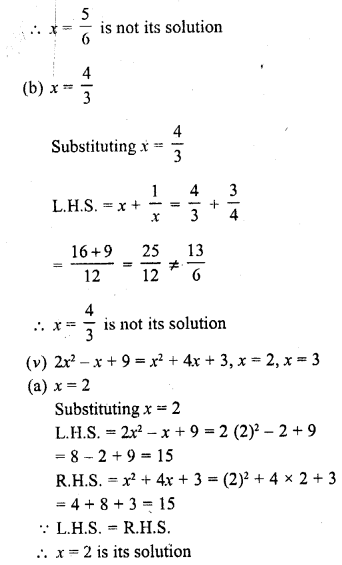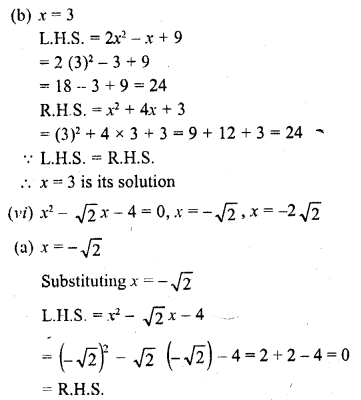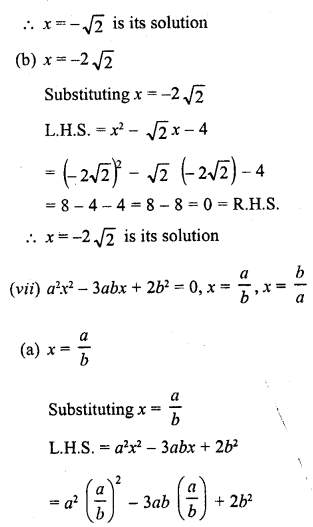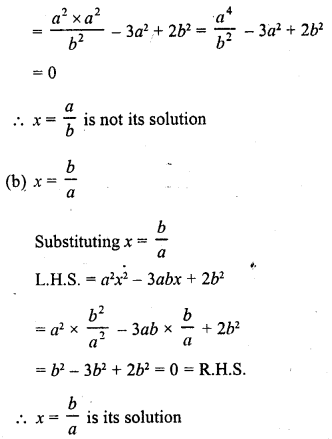Question 3.
In each of the following, find the value of k for which the given value is a solution of the given equation.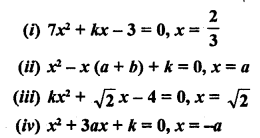Solution: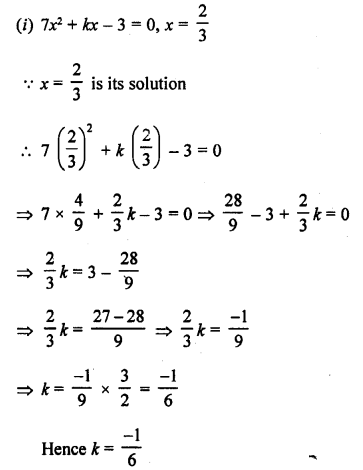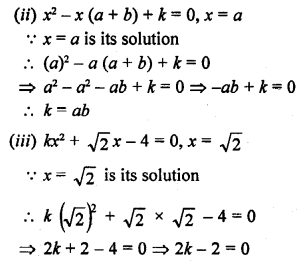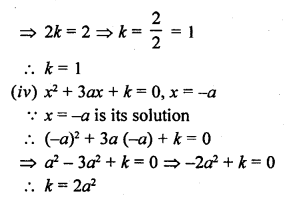Question 4.
Determine, if 3 is a root of the equation given below :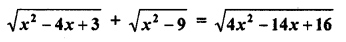Solution: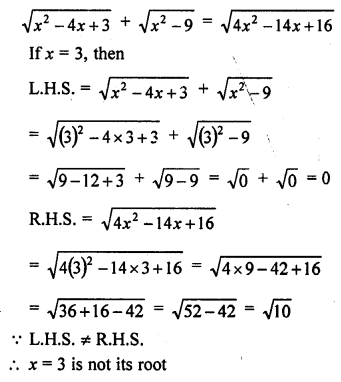Question 5.
If x = $$\frac { 2 }{ 3 }$$ and x = -3 are the roots of the equation ax2 + 7x + b = 0, find the values of a and b.
Solution: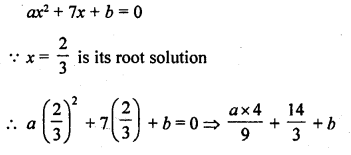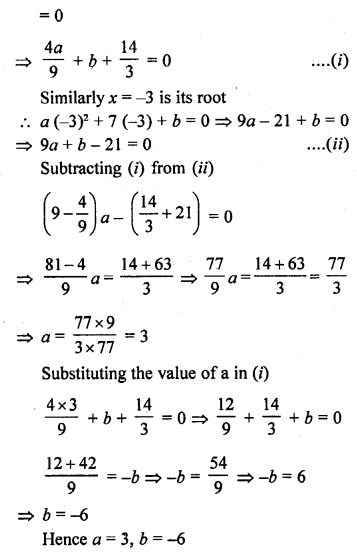Hope given RD Sharma Class 10 Solutions Chapter 4 Quadratic Equations Ex 4.1 are helpful to complete your math homework.

If you have any doubts, please comment below. Learn Insta try to provide online math tutoring for you.